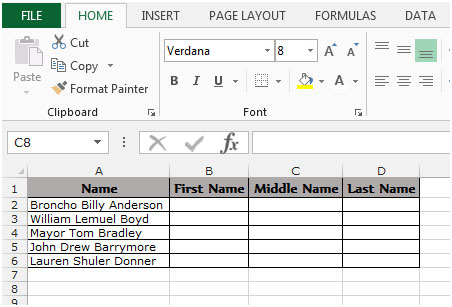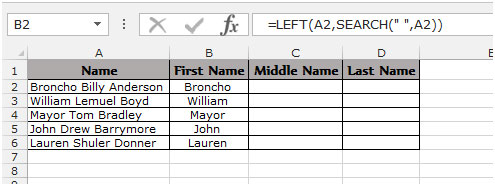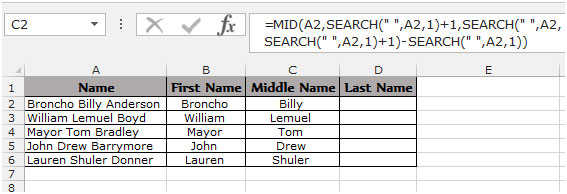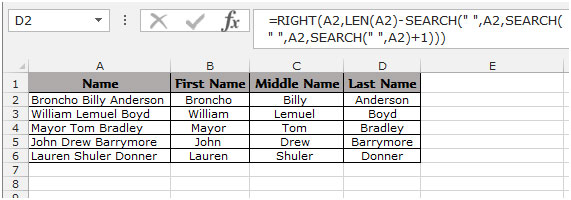Extract the first, middle and last name in Excel 2010

To extract the first, middle and last name we use the formulas “LEFT”, “RIGHT”, “MID”, “LEN”, and “SEARCH” in Excel.

LEFT: Returns the first character(s) in a text string based on the number of characters specified.

Syntax of “LEFT” function: =LEFT (text,[num_chars])

Example:Cell A2 contains the text “Mahesh Kumar Gupta”
=LEFT (A2, 6), function will return “Mahesh”

RIGHT:Return the last character(s) in a text string based on the number of characters specified.

Syntax of “RIGHT” function: =RIGHT (text, [num_chars])

Example:Cell A2 contains the text “Mahesh Kumar Gupta”
=RIGHT (A2, 5), function will return “GUPTA”

MID:Return a specific number of character(s) from a text string, starting at the position specified based on the number of characters specified.

Syntax of “MID” function: =MID (text,start_num,num_chars)

Example:Cell A2 contains the text “Mahesh Kumar Gupta”
=MID (A2, 8, 5), function will return “KUMAR”

LEN:Returns the number of characters in a text string.

Syntax of “LEN” function: =LEN (text)

Example:Cell A2 contains the text “Mahesh Kumar Gupta”
=LEN (A2), function will return 18

SEARCH:The SEARCH function returns the starting position of a text string which it locates from within the text string.

Syntax of “SEARCH” function: =SEARCH (find_text,within_text,[start_num])

Example:Cell A2 contains the text “Mahesh Kumar Gupta”
=SEARCH (“Kumar”, A2, 1), function will return 8

How to separating the names in Excel through formula?

To understand how you can extract the first, middle and last name from the text follow the below mentioned steps:

Example 1: We have a list of Names in Column “A” and we need to pick the First name from the list. We will write the “LEFT” function along with the “SEARCH” function.• Select the Cell B2, write the formula =LEFT (A2, SEARCH (” “, A2)), function will return the first name from the cell A2.
• To Copy the formula in all cells press the key “CTRL + C” and select the cell B3 to B6 and press the key “CTRL + V” on your keyboard.To pick the Middle name from the list. We write the “MID” function along with the “SEARCH” function.

• Select the Cell C2, write the formula =MID(A2,SEARCH(” “,A2,1)+1,SEARCH(” “,A2,SEARCH(” “,A2,1)+1)-SEARCH(” “,A2,1)) it will return the middle name from the cell A2.
• To Copy the formula in all cells press key “CTRL + C” and select the cell C3 to C6 and press key “CTRL + V”on your keyboard.To pick the Last name from the list. We we will use the “RIGHT” function along with the “SEARCH” and “LEN” function.

• Select the Cell D2, write the formula =RIGHT(A2,LEN(A2)-SEARCH(” “,A2,SEARCH(” “,A2,SEARCH(” “,A2)+1))) It will return the last name from the cell A2.
• To Copy the formula in all cells press key “CTRL + C” and select the cell D3 to D6 and press key “CTRL + V” on your keyboard.This is the way we can split names through formula in Microsoft Excel.

To know more find the below examples:-

Extract file name from a path

How to split a full address into 3 or more separate columns

## Users are saying about us...

1.How does one separate out JR’s? eg. John W. Smith Jr.

2.“Try this to find ‘Smith Jr.’
=IF(ISERROR(SEARCH(“” “”,A2,SEARCH(“” “”,A2)+1)),RIGHT(A2,LEN(A2)-SEARCH(“” “”,A2)),RIGHT(A2,LEN(A2)-SEARCH(“” “”,A2,SEARCH(“” “”,A2)+1)))”

3.Michael (micky) Avidan

In order to extract the last name you may try a shorter formula.

=TRIM(RIGHT(SUBSTITUTE(TRIM(A2),” “,REPT(” “,255)),255))

Michael (Micky) Avidan
“Microsoft® Answers” – Wiki author & Forums Moderator
“Microsoft®” MVP – Excel (2009-2014)
ISRAEL

•This is awesome…thank you so much…it compensated for errors from folks with only a first and last name (i.e., not middle name).

•Thankyou……

Any easiest formula for MID name?

•How can extract MID name with this Function Formula “=TRIM(RIGHT(SUBSTITUTE(TRIM(A2),” “,REPT(” “,255)),255))
We can extract FIRST name and LAST name easily with this formula function but middle name not get it. Please help soon.

4.Michael (micky) Avidan

By the Way – if I’m not mistaken the same can be accomplished with the feature: “Text to Columns”.

Michael (Micky) Avidan
“Microsoft® Answers” – Wiki author & Forums Moderator
“Microsoft®” MVP – Excel (2009-2014)
ISRAEL

5.Avnesh chaudhary

plz tell me how to split first and last name if they are combined with any character other than letters. E.G.Avnesh.chaudhary
vishal1mittal
akash#sharma
Prateek_bansal
plz help me..plz

•To extract the last name you can use below mentioned formula
=IF (ISNUMBER (FIND (“,”,A2)),RIGHT(A2,LEN(A2)-FIND(“,”,A2)-1),A2)

To extract the first name use this formula =LEFT (A2, SEARCH (“@”, A2)-1).

•hi can any 1 provide excel links where i can learn with examples please i will be very thankful to you•To extract the name from right you can use below mentioned formula

=IF (ISNUMBER (FIND (“,”,A2)),RIGHT(A2,LEN(A2)-FIND(“,”,A2)-1),A2)

For Example:-
Column A Column B
Names_______________First Names
Bush, George________George
Seinfeld, Jerry_____Jerry
Jordan, Michael_____Michael
Laura_______________Laura
Kate________________Kate

To extract the name from left use this formula =LEFT (A2, SEARCH (“@”, A2)-1)

For Example:-
Column A Column B
Names_______________First Names
type1@gmail.com________type1
type12@gmail.com_____type12
type123@gmail.com_____type123
type1234@gmail.com_____type1234

•Michael (Micky) Avidan

@Nisha Dahawan,
To the best of my knowledge your, suggested, formula does not extract the Last(!) name.
Michael (Micky) Avidan
“Microsoft® Answers” – Wiki author & Forums Moderator
“Microsoft®” MVP – Excel (2009-2014)
ISRAEL

6.i need destination 3 charac in between below mentioned city routing,

YWGYYZLGAYYZYWG
YYZPHLMANPHLYYZ
YYZLHRCAI

7.For getting last name : use

=MID(A2,LOOKUP(1,–((MID(A2,ROW(INDIRECT(“1:” &LEN(A2))),1))=” “),ROW(INDIRECT(“1:” &LEN(A2)))),50)

8.Use Flash Fill, new in Excel 2013, to fill out data based on an example. Flash Fill typically starts working when it recognizes a pattern in your data, and works best when your data has some consistency.

9.excel 1st sheet a,b.given 2 sheet full name given but 1 full name

10.excel 1st sheet a,b.c given 2 sheet full name given but 1 full name then 1 st sheet b number come full name

11.I have a problem extracting compound given names and single names, as well as their middle initial in this order
Larry, Martin Luther S.
Hudson, Mary L.
I used the formula =LEFT(MID(A6,FIND(” “,A6)+1,LEN(A6)),FIND(” “,MID(A6,FIND(” “,A6)+1,LEN(A6)))-1) for first name. OUTPUT was Martin only, I need to add Luther but for Hudson, Mary works just fine,
In the middle initial I used the formula =RIGHT(A23,LEN(A23)-FIND(” “,A23,FIND(” “,A23,FIND(” “,A23)+2))), for Larry, Martin Luther S, output sa Luther S. how can i eliminate Luther but for Hudson, Mary L works just fine,, what should I do? thank you,

12.Tony Clemenger

HELP please: GrahamMaurice – how to obtain two columns = Maurice and the other = Surname = Graham?

Thinking this is simple excel; but I am simple!; best Tony Clemenger;

and I have thousands of them:

CiciullaSerge
CullumCoral
RissonGarry
MelvilleScott
WilliamsRodney
MunroFiona
CraftJamie
BergincPeter
RowlandsScott
KoumidesMel
RavenMark
McGurganJustin
SymonsRycki
KellerEdward

CAN HELP???

•HI Tony,

Here is a formula which can help you split First name and Last name wherein the ONLY delimiter is an Uppercase. This should work on major of the names given in your example list apart from the name like “McGurganJustin” which has 3 uppercase.

Enter the following formula in the designated cells; Considering A1 contains the name: CiciullaSerge

C1 (Last Name) : =MID(A1,MATCH(1,(CODE(MID(A1,ROW(\$1:\$255),1))>=65)*(CODE(MID(A1,ROW(\$2:\$255),1))<90),)+1,255)

PLEASE NOTE: This is an array formula (press CTRL+SHIFT+ENTER)

B1 (First Name) : =SUBSTITUTE(A1,C1,"")

13.Please help. I need to retrieve the middle name with the first character and the last character being in upper case. for instance retrieve “BillY” from broncho billy anderson

•Hi Brian,

We are glad to assist you. Also, we would request you if you have any simple or complicated query, please visit Excel Forum and ask your query to our expert. You will get the solution to your queries in very less time.

Coming back to your query asked by you above, we assume you have “broncho billy anderson” in cell A2. Enter the following formula in B2 to get the desired result as “BillY”.=PROPER(MID(A2,SEARCH(” “,A2,1)+1,SEARCH(” “,A2,SEARCH(” “,A2,1)+1)-SEARCH(” “,A2,1)-2))&””&PROPER(MID(A2,SEARCH(” “,A2,SEARCH(” “,A2,1)+1)-1,1))

Let us know if it helps you to meet your requirement.

If you like the solution, we request you to visit our Facebook page and like us.Best Regards,
Team Excel Tip & Excel Forum

14.how i can make that Name As M k Gupta

•Hi Manoj,

We would request you to please provide the exact criteria to meet your expected result. So our experts can help you in achieving the same.Regards,
Team Excel Tip & Excel Forum

15.Just don’t get in all this mess just simply copy paste the formula given below in formula bar:

=LEFT(A2, SEARCH (” “, A2)-1)

=IFERROR(MID(A2,SEARCH(” “,A2,1)+1,SEARCH(” “,A2,SEARCH(” “,A2,1)+1)-SEARCH(” “,A2,1)),””)

=IFERROR(REPLACE(A2,1,SEARCH(“^”,SUBSTITUTE(A2,” “,”^”,LEN(A2)-LEN(SUBSTITUTE(A2,” “,””)))),””),””)

Recommended only on 2010 and above

16.None of these work………you either get a Value! or Name! error message……………….

17.Hi,

this formulae is used for three names.

i have a requirements of 4 names. how to split them in 4 columns?

for example, i have First Name, Second Name, Third Name and Forth Name in one column combined all together. i want to split them in four columns.

Regards.

•Assuming you have “First Second Third Fourth” in cell A1 and as per your request you want to split them as First, Second, Third & Fourth names in individual columns respectively so the formula would be..

To extract
First Name –> “=LEFT(A1,SEARCH(” “,A1,1)-1)”
Second Name –> “=MID(A1,SEARCH(” “,A1,1)+1,(SEARCH(” “,A1,SEARCH(” “,A1,1)+1)-1)-SEARCH(” “,A1,1))”
Third Name —> “=MID(A1,SEARCH(” “,A1,SEARCH(” “,A1,1)+1)+1,SEARCH(” “,A1,SEARCH(” “,A1,SEARCH(” “,A1,1)+1)+1)-SEARCH(” “,A1,SEARCH(” “,A1,1)+1)-1)”
Fourth Name —> =RIGHT(A1,LEN(A1)-SEARCH(” “,A1,SEARCH(” “,A1,SEARCH(” “,A1,1)+1)+1))

Hope this is what you were looking for.

Happy Learning,

18.•Hi Samson,

We assume that you have all emails like this.

To extract left name “wendy”, use the following formula –> “=LEFT(“wendy.poulton@eskom.co.za”,SEARCH(“.”,”wendy.poulton@eskom.co.za”,1)-1)”

To extract the last name “poulton”, use the following formula –> “=MID(“wendy.poulton@eskom.co.za”,SEARCH(“.”,”wendy.poulton@eskom.co.za”,1)+1,SEARCH(“@”,”wendy.poulton@eskom.co.za”,1)-SEARCH(“.”,”wendy.poulton@eskom.co.za”,1)-1)”.

You may find both formulas lengthy but you can replace the email id with the cell reference and it will extract the first and last name from email address.

Also, we will request you to please visit http://www.excelforum.com in case you have any Excel/VBA query. You can ask our experts and get the instant solutions for your queries.

Thanks,

19.Hi All, i have a list of names Ex-

CA
PA-US-PA
PA-US-PA
PA-US-PA
IN
PA
however, my friend is asking me to put one formula for all but the outcome should only be “US”. in this case single words will remain same like CN,IN and for 3 words (PA-US-PA) the result should be only US.
Pls help me with this i m nt able to put a logic here.

•Hi Ragini,

As per requirement, you don’t want to extract the names however you need to use the logical statement here which will check if single word, then return as it is otherwise look for “-US*” and return “US” as output in the cell. If this is the case, following is the formula will help you to retrieve expected output.

=IF(COUNTIF(A1,”*-US-*”)=1,”US”,A1)

We assumed you have data in column A from cell A1 to A6. Put the above formula in corresponding cell (B1 to down) and it will return what you looking for.

Also, we’d recommend you to login on our official website for your simple or complicated Excel/VBA query and get instant solution for the same.

Cheers!

20.How about you have 2nd name
ex: “CHLOE RAYNE” MENDOZA DE LEON

John Andrew S. Curry

21.Hi i need to extract number from below data

‘as12asd21mis45asdds2′ Result 1221452
asafas1457 Result 1457

22.how to remove space and country code from below data

145 2145145 result 1452145145
9987 954748 result 9987954748
9945 45 4878 result 9945454878
’91 9987 125445 result 9987125445

23.Deepak Maurya

Hallo

Please tell me that how can do the 3 characters in find last name
such as: Deepak Kumar Maurya then find Last name means Maurya text find.